# quebec.pdf

1. Jun 2023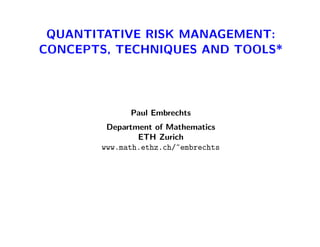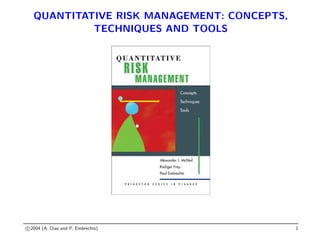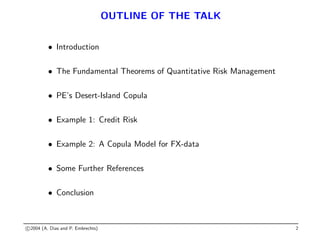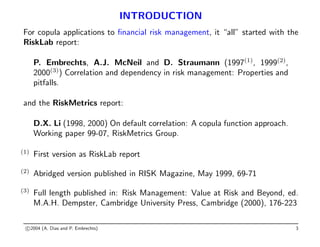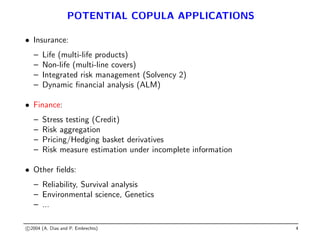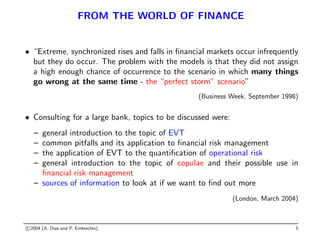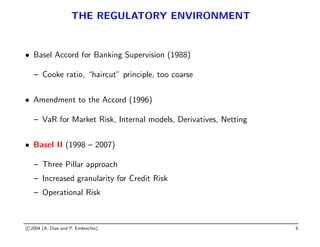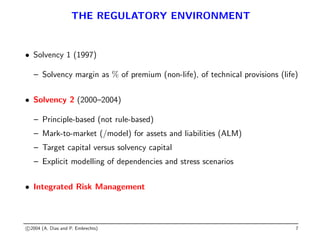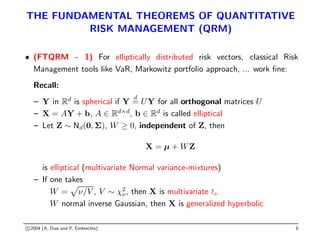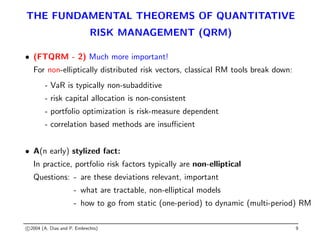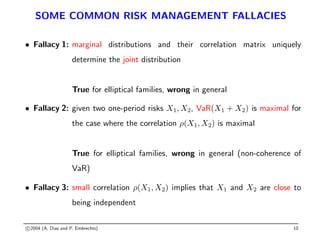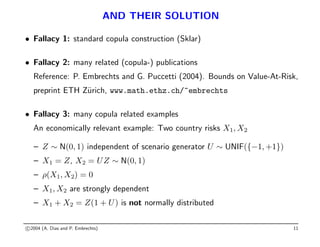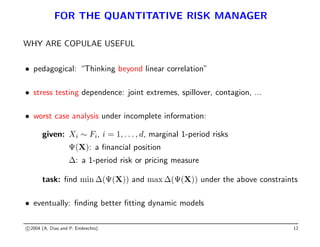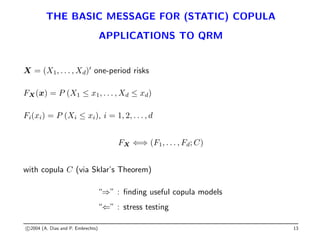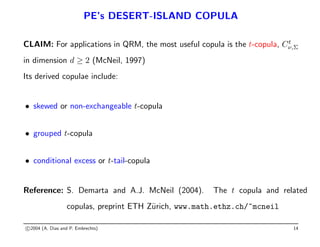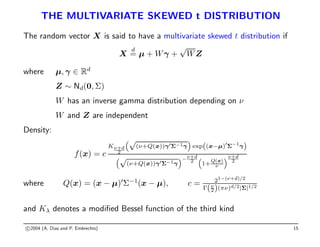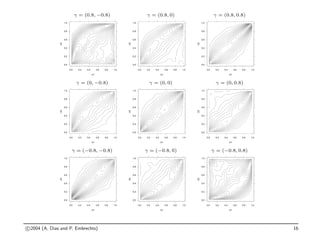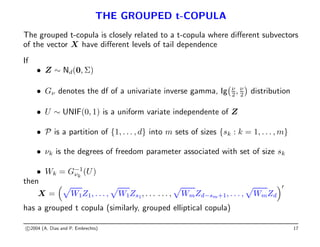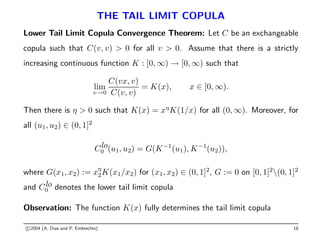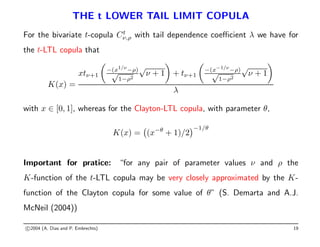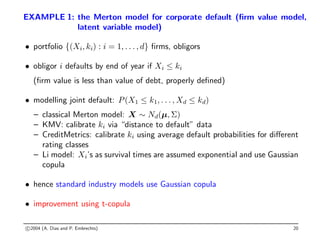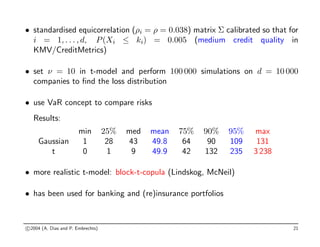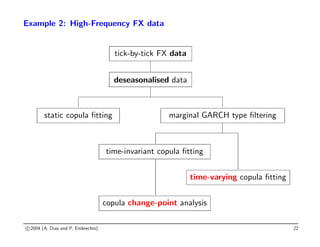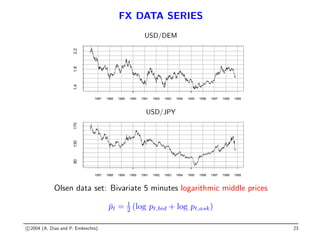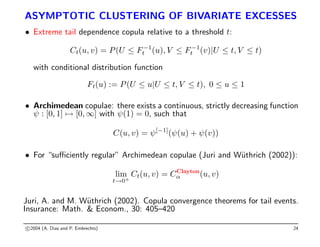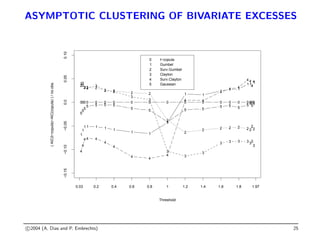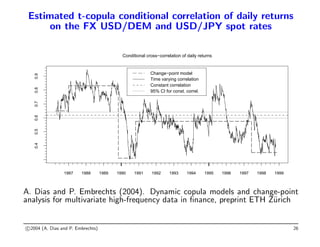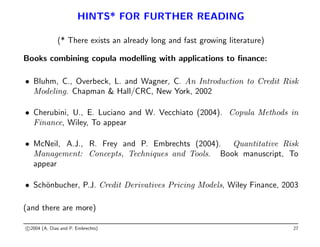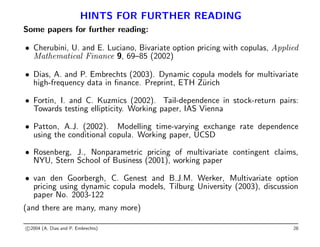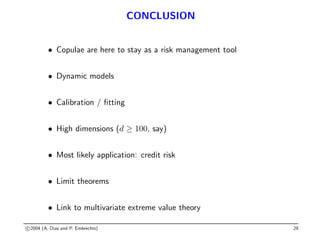1 von 30

### quebec.pdf

• 1. QUANTITATIVE RISK MANAGEMENT: CONCEPTS, TECHNIQUES AND TOOLS* Paul Embrechts Department of Mathematics ETH Zurich www.math.ethz.ch/~embrechts
• 2. QUANTITATIVE RISK MANAGEMENT: CONCEPTS, TECHNIQUES AND TOOLS c 2004 (A. Dias and P. Embrechts) 1
• 3. OUTLINE OF THE TALK • Introduction • The Fundamental Theorems of Quantitative Risk Management • PE’s Desert-Island Copula • Example 1: Credit Risk • Example 2: A Copula Model for FX-data • Some Further References • Conclusion c 2004 (A. Dias and P. Embrechts) 2
• 4. INTRODUCTION For copula applications to financial risk management, it “all” started with the RiskLab report: P. Embrechts, A.J. McNeil and D. Straumann (1997(1) , 1999(2) , 2000(3) ) Correlation and dependency in risk management: Properties and pitfalls. and the RiskMetrics report: D.X. Li (1998, 2000) On default correlation: A copula function approach. Working paper 99-07, RiskMetrics Group. (1) First version as RiskLab report (2) Abridged version published in RISK Magazine, May 1999, 69-71 (3) Full length published in: Risk Management: Value at Risk and Beyond, ed. M.A.H. Dempster, Cambridge University Press, Cambridge (2000), 176-223 c 2004 (A. Dias and P. Embrechts) 3
• 5. POTENTIAL COPULA APPLICATIONS • Insurance: – Life (multi-life products) – Non-life (multi-line covers) – Integrated risk management (Solvency 2) – Dynamic financial analysis (ALM) • Finance: – Stress testing (Credit) – Risk aggregation – Pricing/Hedging basket derivatives – Risk measure estimation under incomplete information • Other fields: – Reliability, Survival analysis – Environmental science, Genetics – ... c 2004 (A. Dias and P. Embrechts) 4
• 6. FROM THE WORLD OF FINANCE • “Extreme, synchronized rises and falls in financial markets occur infrequently but they do occur. The problem with the models is that they did not assign a high enough chance of occurrence to the scenario in which many things go wrong at the same time - the “perfect storm” scenario” (Business Week, September 1998) • Consulting for a large bank, topics to be discussed were: – general introduction to the topic of EVT – common pitfalls and its application to financial risk management – the application of EVT to the quantification of operational risk – general introduction to the topic of copulae and their possible use in financial risk management – sources of information to look at if we want to find out more (London, March 2004) c 2004 (A. Dias and P. Embrechts) 5
• 7. THE REGULATORY ENVIRONMENT • Basel Accord for Banking Supervision (1988) – Cooke ratio, “haircut” principle, too coarse • Amendment to the Accord (1996) – VaR for Market Risk, Internal models, Derivatives, Netting • Basel II (1998 – 2007) – Three Pillar approach – Increased granularity for Credit Risk – Operational Risk c 2004 (A. Dias and P. Embrechts) 6
• 8. THE REGULATORY ENVIRONMENT • Solvency 1 (1997) – Solvency margin as % of premium (non-life), of technical provisions (life) • Solvency 2 (2000–2004) – Principle-based (not rule-based) – Mark-to-market (/model) for assets and liabilities (ALM) – Target capital versus solvency capital – Explicit modelling of dependencies and stress scenarios • Integrated Risk Management c 2004 (A. Dias and P. Embrechts) 7
• 9. THE FUNDAMENTAL THEOREMS OF QUANTITATIVE RISK MANAGEMENT (QRM) • (FTQRM - 1) For elliptically distributed risk vectors, classical Risk Management tools like VaR, Markowitz portfolio approach, ... work fine: Recall: – Y in Rd is spherical if Y d = UY for all orthogonal matrices U – X = AY + b, A ∈ Rd×d , b ∈ Rd is called elliptical – Let Z ∼ Nd(0, Σ), W ≥ 0, independent of Z, then X = µ + WZ is elliptical (multivariate Normal variance-mixtures) – If one takes W = p ν/V , V ∼ χ2 ν, then X is multivariate tν W normal inverse Gaussian, then X is generalized hyperbolic c 2004 (A. Dias and P. Embrechts) 8
• 10. THE FUNDAMENTAL THEOREMS OF QUANTITATIVE RISK MANAGEMENT (QRM) • (FTQRM - 2) Much more important! For non-elliptically distributed risk vectors, classical RM tools break down: - VaR is typically non-subadditive - risk capital allocation is non-consistent - portfolio optimization is risk-measure dependent - correlation based methods are insufficient • A(n early) stylized fact: In practice, portfolio risk factors typically are non-elliptical Questions: - are these deviations relevant, important - what are tractable, non-elliptical models - how to go from static (one-period) to dynamic (multi-period) RM c 2004 (A. Dias and P. Embrechts) 9
• 11. SOME COMMON RISK MANAGEMENT FALLACIES • Fallacy 1: marginal distributions and their correlation matrix uniquely determine the joint distribution True for elliptical families, wrong in general • Fallacy 2: given two one-period risks X1, X2, VaR(X1 + X2) is maximal for the case where the correlation ρ(X1, X2) is maximal True for elliptical families, wrong in general (non-coherence of VaR) • Fallacy 3: small correlation ρ(X1, X2) implies that X1 and X2 are close to being independent c 2004 (A. Dias and P. Embrechts) 10
• 12. AND THEIR SOLUTION • Fallacy 1: standard copula construction (Sklar) • Fallacy 2: many related (copula-) publications Reference: P. Embrechts and G. Puccetti (2004). Bounds on Value-At-Risk, preprint ETH Zürich, www.math.ethz.ch/~embrechts • Fallacy 3: many copula related examples An economically relevant example: Two country risks X1, X2 – Z ∼ N(0, 1) independent of scenario generator U ∼ UNIF({−1, +1}) – X1 = Z, X2 = UZ ∼ N(0, 1) – ρ(X1, X2) = 0 – X1, X2 are strongly dependent – X1 + X2 = Z(1 + U) is not normally distributed c 2004 (A. Dias and P. Embrechts) 11
• 13. FOR THE QUANTITATIVE RISK MANAGER WHY ARE COPULAE USEFUL • pedagogical: “Thinking beyond linear correlation” • stress testing dependence: joint extremes, spillover, contagion, ... • worst case analysis under incomplete information: given: Xi ∼ Fi, i = 1, . . . , d, marginal 1-period risks Ψ(X): a financial position ∆: a 1-period risk or pricing measure task: find min ∆(Ψ(X)) and max ∆(Ψ(X)) under the above constraints • eventually: finding better fitting dynamic models c 2004 (A. Dias and P. Embrechts) 12
• 14. THE BASIC MESSAGE FOR (STATIC) COPULA APPLICATIONS TO QRM X = (X1, . . . , Xd)0 one-period risks FX(x) = P (X1 ≤ x1, . . . , Xd ≤ xd) Fi(xi) = P (Xi ≤ xi), i = 1, 2, . . . , d FX ⇐⇒ (F1, . . . , Fd; C) with copula C (via Sklar’s Theorem) “⇒” : finding useful copula models “⇐” : stress testing c 2004 (A. Dias and P. Embrechts) 13
• 15. PE’s DESERT-ISLAND COPULA CLAIM: For applications in QRM, the most useful copula is the t-copula, Ct ν,Σ in dimension d ≥ 2 (McNeil, 1997) Its derived copulae include: • skewed or non-exchangeable t-copula • grouped t-copula • conditional excess or t-tail-copula Reference: S. Demarta and A.J. McNeil (2004). The t copula and related copulas, preprint ETH Zürich, www.math.ethz.ch/~mcneil c 2004 (A. Dias and P. Embrechts) 14
• 16. THE MULTIVARIATE SKEWED t DISTRIBUTION The random vector X is said to have a multivariate skewed t distribution if X d = µ + Wγ + √ WZ where µ, γ ∈ Rd Z ∼ Nd(0, Σ) W has an inverse gamma distribution depending on ν W and Z are independent Density: f(x) = c Kν+d 2 “√ (ν+Q(x))γ0Σ−1γ ” exp((x−µ)0 Σ−1 γ) “√ (ν+Q(x))γ0Σ−1γ ”−ν+d 2 “ 1+ Q(x) ν ”ν+d 2 where Q(x) = (x − µ)0 Σ−1 (x − µ), c = 21−(ν+d)/2 Γ(ν 2)(πν)d/2|Σ|1/2 and Kλ denotes a modified Bessel function of the third kind c 2004 (A. Dias and P. Embrechts) 15
• 17. γ = (0.8, −0.8) 0.0 0.2 0.4 0.6 0.8 1.0 0.0 0.2 0.4 0.6 0.8 1.0 U1 U2 γ = (0.8, 0) 0.0 0.2 0.4 0.6 0.8 1.0 0.0 0.2 0.4 0.6 0.8 1.0 U1 U2 γ = (0.8, 0.8) 0.0 0.2 0.4 0.6 0.8 1.0 0.0 0.2 0.4 0.6 0.8 1.0 U1 U2 γ = (0, −0.8) 0.0 0.2 0.4 0.6 0.8 1.0 0.0 0.2 0.4 0.6 0.8 1.0 U1 U2 γ = (0, 0) 0.0 0.2 0.4 0.6 0.8 1.0 0.0 0.2 0.4 0.6 0.8 1.0 U1 U2 γ = (0, 0.8) 0.0 0.2 0.4 0.6 0.8 1.0 0.0 0.2 0.4 0.6 0.8 1.0 U1 U2 γ = (−0.8, −0.8) 0.0 0.2 0.4 0.6 0.8 1.0 0.0 0.2 0.4 0.6 0.8 1.0 U1 U2 γ = (−0.8, 0) 0.0 0.2 0.4 0.6 0.8 1.0 0.0 0.2 0.4 0.6 0.8 1.0 U1 U2 γ = (−0.8, 0.8) 0.0 0.2 0.4 0.6 0.8 1.0 0.0 0.2 0.4 0.6 0.8 1.0 U1 U2 c 2004 (A. Dias and P. Embrechts) 16
• 18. THE GROUPED t-COPULA The grouped t-copula is closely related to a t-copula where different subvectors of the vector X have different levels of tail dependence If • Z ∼ Nd(0, Σ) • Gν denotes the df of a univariate inverse gamma, Ig ν 2, ν 2 distribution • U ∼ UNIF(0, 1) is a uniform variate independente of Z • P is a partition of {1, . . . , d} into m sets of sizes {sk : k = 1, . . . , m} • νk is the degrees of freedom parameter associated with set of size sk • Wk = G−1 νk (U) then X = p W1Z1, . . . , p W1Zs1, . . . . . . , p WmZd−sm+1, . . . , p WmZd 0 has a grouped t copula (similarly, grouped elliptical copula) c 2004 (A. Dias and P. Embrechts) 17
• 19. THE TAIL LIMIT COPULA Lower Tail Limit Copula Convergence Theorem: Let C be an exchangeable copula such that C(v, v) 0 for all v 0. Assume that there is a strictly increasing continuous function K : [0, ∞) → [0, ∞) such that lim v→0 C(vx, v) C(v, v) = K(x), x ∈ [0, ∞). Then there is η 0 such that K(x) = xη K(1/x) for all (0, ∞). Moreover, for all (u1, u2) ∈ (0, 1]2 Clo 0 (u1, u2) = G(K−1 (u1), K−1 (u2)), where G(x1, x2) := xη 2K(x1/x2) for (x1, x2) ∈ (0, 1]2 , G := 0 on [0, 1]2 (0, 1]2 and Clo 0 denotes the lower tail limit copula Observation: The function K(x) fully determines the tail limit copula c 2004 (A. Dias and P. Embrechts) 18
• 20. THE t LOWER TAIL LIMIT COPULA For the bivariate t-copula Ct ν,ρ with tail dependence coefficient λ we have for the t-LTL copula that K(x) = xtν+1 −(x1/ν −ρ) √ 1−ρ2 √ ν + 1 + tν+1 −(x−1/ν −ρ) √ 1−ρ2 √ ν + 1 λ with x ∈ [0, 1], whereas for the Clayton-LTL copula, with parameter θ, K(x) = (x−θ + 1)/2 −1/θ Important for pratice: “for any pair of parameter values ν and ρ the K-function of the t-LTL copula may be very closely approximated by the K- function of the Clayton copula for some value of θ” (S. Demarta and A.J. McNeil (2004)) c 2004 (A. Dias and P. Embrechts) 19
• 21. EXAMPLE 1: the Merton model for corporate default (firm value model, latent variable model) • portfolio {(Xi, ki) : i = 1, . . . , d} firms, obligors • obligor i defaults by end of year if Xi ≤ ki (firm value is less than value of debt, properly defined) • modelling joint default: P(X1 ≤ k1, . . . , Xd ≤ kd) – classical Merton model: X ∼ Nd(µ, Σ) – KMV: calibrate ki via “distance to default” data – CreditMetrics: calibrate ki using average default probabilities for different rating classes – Li model: Xi’s as survival times are assumed exponential and use Gaussian copula • hence standard industry models use Gaussian copula • improvement using t-copula c 2004 (A. Dias and P. Embrechts) 20
• 22. • standardised equicorrelation (ρi = ρ = 0.038) matrix Σ calibrated so that for i = 1, . . . , d, P(Xi ≤ ki) = 0.005 (medium credit quality in KMV/CreditMetrics) • set ν = 10 in t-model and perform 100 000 simulations on d = 10 000 companies to find the loss distribution • use VaR concept to compare risks Results: min 25% med mean 75% 90% 95% max Gaussian 1 28 43 49.8 64 90 109 131 t 0 1 9 49.9 42 132 235 3 238 • more realistic t-model: block-t-copula (Lindskog, McNeil) • has been used for banking and (re)insurance portfolios c 2004 (A. Dias and P. Embrechts) 21
• 23. Example 2: High-Frequency FX data tick-by-tick FX data deseasonalised data static copula fitting marginal GARCH type filtering time-invariant copula fitting time-varying copula fitting copula change-point analysis c 2004 (A. Dias and P. Embrechts) 22
• 24. FX DATA SERIES USD/DEM 1987 1988 1989 1990 1991 1992 1993 1994 1995 1996 1997 1998 1999 1.4 1.8 2.2 USD/JPY 1987 1988 1989 1990 1991 1992 1993 1994 1995 1996 1997 1998 1999 90 130 170 Olsen data set: Bivariate 5 minutes logarithmic middle prices p̄t = 1 2 (log pt,bid + log pt,ask) c 2004 (A. Dias and P. Embrechts) 23
• 25. ASYMPTOTIC CLUSTERING OF BIVARIATE EXCESSES • Extreme tail dependence copula relative to a threshold t: Ct(u, v) = P(U ≤ F−1 t (u), V ≤ F−1 t (v)|U ≤ t, V ≤ t) with conditional distribution function Ft(u) := P(U ≤ u|U ≤ t, V ≤ t), 0 ≤ u ≤ 1 • Archimedean copulae: there exists a continuous, strictly decreasing function ψ : [0, 1] 7→ [0, ∞] with ψ(1) = 0, such that C(u, v) = ψ[−1] (ψ(u) + ψ(v)) • For “sufficiently regular” Archimedean copulae (Juri and Wüthrich (2002)): lim t→0+ Ct(u, v) = CClayton α (u, v) Juri, A. and M. Wüthrich (2002). Copula convergence theorems for tail events. Insurance: Math. Econom., 30: 405–420 c 2004 (A. Dias and P. Embrechts) 24
• 26. ASYMPTOTIC CLUSTERING OF BIVARIATE EXCESSES 1 1 1 1 1 1 1 1 1 1 1 1 1 1 1 1 11 1 Threshold ( AIC(t−copula)−AIC(copula) ) / no.obs. −0.15 −0.10 −0.05 0.0 0.05 0.10 22 2 2 2 2 2 2 2 2 2 2 2 2 2 2 2 2 2 33 3 3 3 3 3 3 3 3 3 3 3 3 3 3 33 3 4 4 4 4 4 4 4 4 4 4 4 4 4 4 4 4 4 4 4 5 5 5 5 5 5 5 5 5 5 5 5 5 5 5 5 5 5 5 000 0 0 0 0 0 0 0 0 0 0 0 0 0 000 0 t−copula 1 Gumbel 2 Surv.Gumbel 3 Clayton 4 Surv.Clayton 5 Gaussian 0.03 0.2 0.4 0.6 0.8 1 1.2 1.4 1.6 1.8 1.97 c 2004 (A. Dias and P. Embrechts) 25
• 27. Estimated t-copula conditional correlation of daily returns on the FX USD/DEM and USD/JPY spot rates Conditional cross−correlation of daily returns 1987 1988 1989 1990 1991 1992 1993 1994 1995 1996 1997 1998 1999 0.4 0.5 0.6 0.7 0.8 0.9 Change−point model Time varying correlation Constant correlation 95% CI for const. correl. A. Dias and P. Embrechts (2004). Dynamic copula models and change-point analysis for multivariate high-frequency data in finance, preprint ETH Zürich c 2004 (A. Dias and P. Embrechts) 26
• 28. HINTS* FOR FURTHER READING (* There exists an already long and fast growing literature) Books combining copula modelling with applications to finance: • Bluhm, C., Overbeck, L. and Wagner, C. An Introduction to Credit Risk Modeling. Chapman Hall/CRC, New York, 2002 • Cherubini, U., E. Luciano and W. Vecchiato (2004). Copula Methods in Finance, Wiley, To appear • McNeil, A.J., R. Frey and P. Embrechts (2004). Quantitative Risk Management: Concepts, Techniques and Tools. Book manuscript, To appear • Schönbucher, P.J. Credit Derivatives Pricing Models, Wiley Finance, 2003 (and there are more) c 2004 (A. Dias and P. Embrechts) 27
• 29. HINTS FOR FURTHER READING Some papers for further reading: • Cherubini, U. and E. Luciano, Bivariate option pricing with copulas, Applied Mathematical Finance 9, 69–85 (2002) • Dias, A. and P. Embrechts (2003). Dynamic copula models for multivariate high-frequency data in finance. Preprint, ETH Zürich • Fortin, I. and C. Kuzmics (2002). Tail-dependence in stock-return pairs: Towards testing ellipticity. Working paper, IAS Vienna • Patton, A.J. (2002). Modelling time-varying exchange rate dependence using the conditional copula. Working paper, UCSD • Rosenberg, J., Nonparametric pricing of multivariate contingent claims, NYU, Stern School of Business (2001), working paper • van den Goorbergh, C. Genest and B.J.M. Werker, Multivariate option pricing using dynamic copula models, Tilburg University (2003), discussion paper No. 2003-122 (and there are many, many more) c 2004 (A. Dias and P. Embrechts) 28
• 30. CONCLUSION • Copulae are here to stay as a risk management tool • Dynamic models • Calibration / fitting • High dimensions (d ≥ 100, say) • Most likely application: credit risk • Limit theorems • Link to multivariate extreme value theory c 2004 (A. Dias and P. Embrechts) 29